## A 0.106-A current is charging a capacitor that has square plates 6.00 cm on each side. The plate separation is 4.00 mm. (a) Find the time ra

Question

A 0.106-A current is charging a capacitor that has square plates 6.00 cm on each side. The plate separation is 4.00 mm. (a) Find the time rate of change of electric flux between the plates.

in progress 0
6 months 2021-08-05T21:33:23+00:00 1 Answers 8 views 0

## Answers ( )

The time rate of change of flux is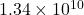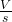Explanation:

Given :

Current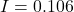A

Area of plate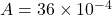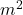Plate separation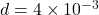m

(A)

First find the capacitance of capacitor,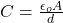Where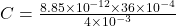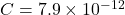F

But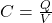Where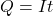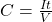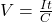Now differentiate above equation wrt. time,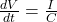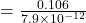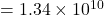Therefore, the time rate of change of flux is